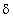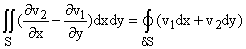## 10.1 Green's Theorem

This theorem is an application of the fundamental theorem of calculus to integrating a certain combinations of derivatives over a plane. It can be proven easily for rectangular and triangular regions.Ā Both sides of its equality are finitely additive so that the result holds for any planar region practically allĀ of which can be divided into triangles and rectangles.Ā This proves the theorem for reasonably shaped regions. Its generalization to non-planar surfaces (proved directly from it by using the finite additivity of both sides) is Stokes' Theorem described below.
Its formal statement is as follows:

Let S be a sufficiently nice region in the plane, and letS be its boundary; then we havewhere the boundary,S is traversed counterclockwise on its outside cycle, (and clockwise on any internal cycles as you can verify using zippers.)

Meaning of this theorem: Green's Theorem is a form that the fundamental theorem of calculus takes in the context of integrals over planar regions.

Proof